# MCQ in DC Circuits Part 12 | REE Board Exam

(Last Updated On: November 13, 2020)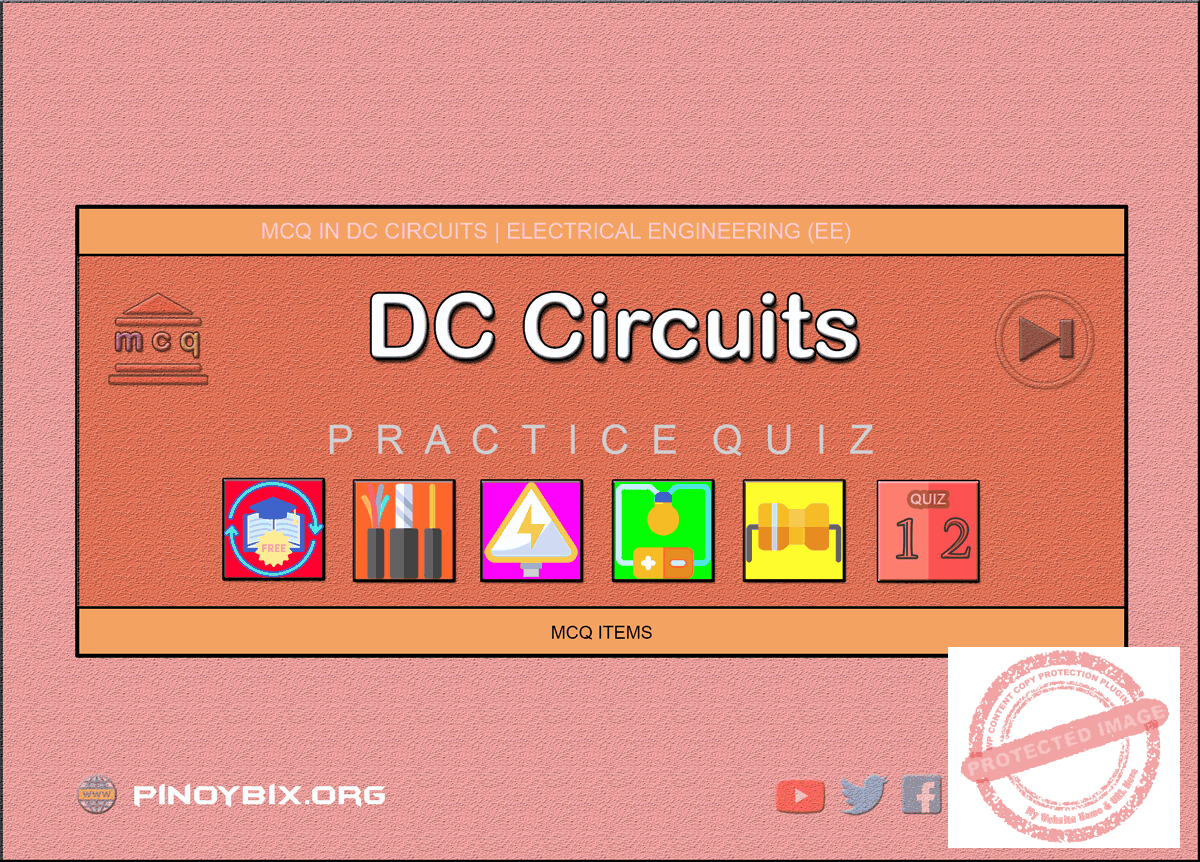This is the Multiple Choice Questions Part 12 of the Series in DC Circuits as one of the Electrical Engineering topic. In Preparation for the REE Board Exam make sure to expose yourself and familiarize in each and every questions compiled here taken from various sources including but not limited to past Board Exam Questions in Electrical Engineering field, Electrical Engineering Books, Journals and other Electrical Engineering References.

#### Continue Practice Exam Test Questions Part 12 of the Series

NETWORK THEOREMS

Choose the letter of the best answer in each questions.

551.  Find the total resistance Rin is in the circuit shown.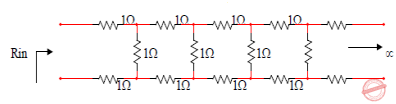A.  1 + √3

B.   (1 – √5)/3

C.   (-1 + √5)/2

D.  none of these

Solution:

552.  What is the value of i1?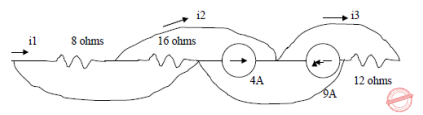A.  0

B.  –6

C.  6

D.  none of these

Solution:

553.  Find Ix in the circuit shown.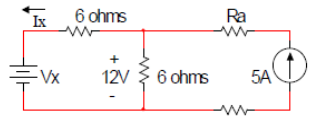A.  3A

B.  –3 A

C.  0

D.  none of these

Solution:

554.  Find value of R in the given circuit.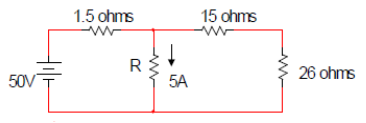A.  8.2 Ω

B.  6 Ω

C.  10 Ω

D.  none of these

Solution:

555.  The voltage V in the figure  always equal to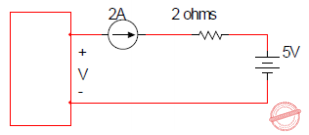A.  9 V

B.  5 V

C.  1 V

D.  none of these

Solution:

556.  Find V in the circuit shown.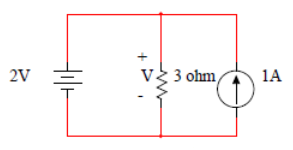A.  2 V

B.  3 V

C.  1 V

D.  none of these

Solution:

557.  Find V in the circuit shown.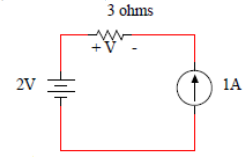A.  – 3 V

B.  +3 V

C.  2 V

D.  none of these

Solution:

558.  Find V in the circuit shown.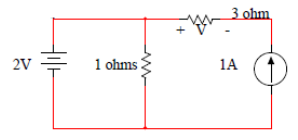A.  + 3 V

B.  – 3 V

C.  2 V

D.  none of these

Solution:

559.  Determine VX of this circuit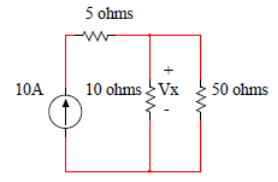A.  42.2 V

B.  83.3 V

C.  97.3   V

D.  103  V

Solution:

560.  Find voltage eo in the fig shown.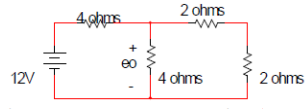A.  2 V

B.  4/3 V

C.  4 V

D.  8 V

Solution:

561.  Find VX in the circuit shown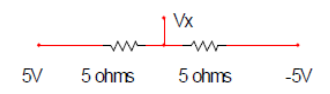A.  2.5 V

B.  -2.5 V

C.  0 V

D.  10 V

Solution:

562.  Find voltage eo in the fig shown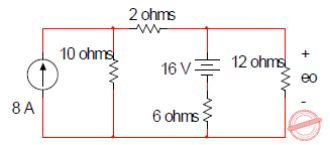A.  48 V

B.  24 V

C.  36 V

D.  28 V

Solution:

563.  The voltage v(t) is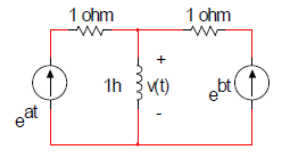A.  eat – e-bt

B.  eat + ebt

C.  aeat – bebt

D.  aeat + bebt

Solution:

564.  Find current through 5 Ω resistor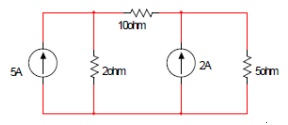A.  0

B.  2 A

C.  3 A

D.  7 A

Solution:

565.  Find Vxy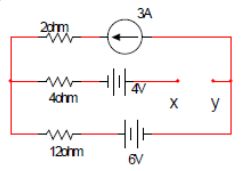A.  10 V

B.  46 V

C.  13 V

D.  58 V

Solution:

566.  What is VAB?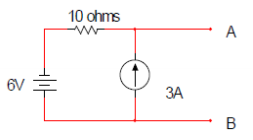A.  3V

B.  54V

C.  24 V

D.  none of these

Solution:

567.  What is Vxy?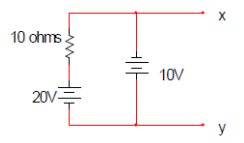A.  20 V

B.  30 V

C.  –10 V

D.  10 V

Solution:

568.  In  the  circuit  of  the  given  figure.  The  value  of  the voltage source E is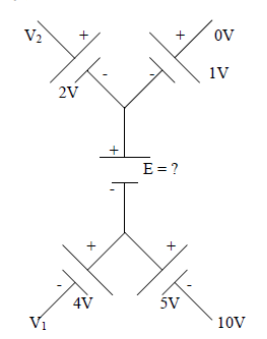A.  –16 V

B.  4 V

C.  –6 V

D.  16 V

Solution:

569.  Find i2 in the figure shown.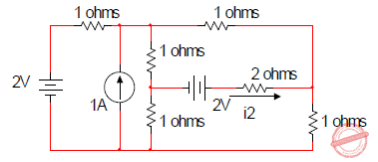A.  4 A

B.  2/3 A

C.  -2/3 A

D.  none of these

Solution:

570.  When a resistor R is connected to a current source, it consumes a power of 18 W. When the same R is connected  to  a  voltage  source  having  same magnitude  as  the  current  source,  the  power absorbed  by  R  is  4.5  W.  The  magnitude  of  the current source & value of R are

A.  √18 A & 1 ohm

B.  3 A & 2 ohms

C.  1 A & 18 ohms

D.  6 A & 0.5 ohms

Solution:

571.  In the circuit shown in the figure. If I = 2, then the value of the battery voltage V will be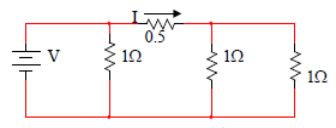A.  5 V

B.  3 V

C.  2 V

D.  1 V

Solution:

572.  Find E and I in the figure shown.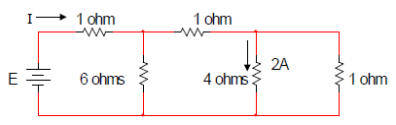A.  I = 13 A and E = 31 V

B.  I = 31 A and E = 13V

C.  E = 31 V and I = 31A

D.  none of these

Solution:

573.  Find the voltage across the terminals a and b.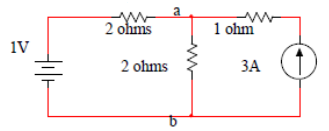A.  0.5 V

B.  3.0 V

C.  3.5 V

D.  4.0 V

Solution:

574.  What  is  the  current  supplied  by  1  V  source  when each resistance is 1 ohm?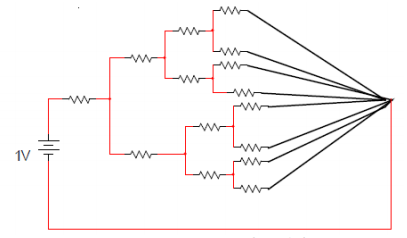A.  8/15 A

B.  15/4 A

C.  4/15   A

D.  none of these

Solution:

575.  The voltage V is equal to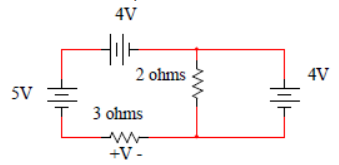A.  3 V

B.  –3 V

C.  5 V

D.  none of these

Solution:

576.  The voltage across 15 ohms resistor is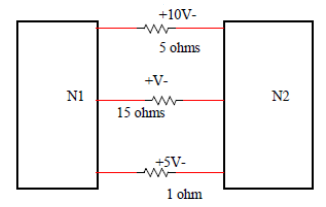A.  -105 V

B.  +105 V

C.  –15 V

D.  + 15 V

Solution:

577.  In the circuit of the given figure. The current I will be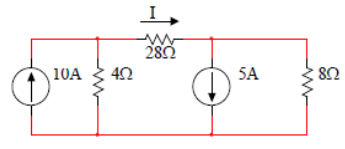A.  1 A

B.  2 A

C.  4 A

D.  8 A

Solution:

578.  In the circuit shown in  the given  figure, the potential difference V2 – V1 is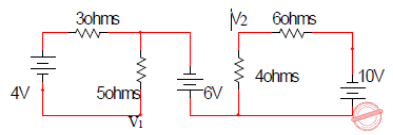A.  –4.5 V

B.  0

C.  4.5 V

D.  6 V

Solution:

579.  Find V in the figure shown.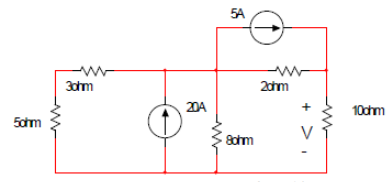A.  56.25 V

B.  85 V

C.  40 V

D.  none of these

Solution:

580.  What is VA?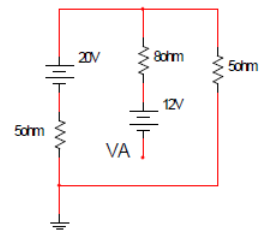A.  -2 V

B.  2 V

C.   -4 V

D.  4 V

Solution:

581.  What is the value of I4 in the fig shown?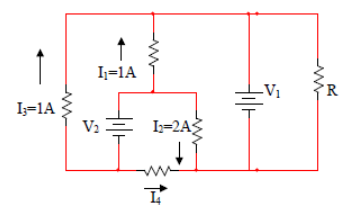A.  –4 A

B.  –2 A

C.  known only if V1, V2 and R are known

D.  known only if V1, V2 are known

Solution:

582.  If the voltage of each source in the given network is doubled,  then  which  of  the  following  statement would be true?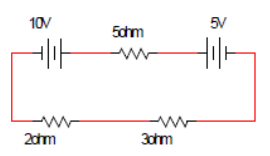1.  Current flowing in the network will be doubled

2.  Voltages across each resistor will be doubled

3.  Power  absorbed  by  each  resistor  will  be doubled

4.  Power  delivered  by  each  source  will  be doubled

A.  1, 2, 3, 4

B.  1, 2

C.  2, 3

D.  1, 3, 4

Solution:

583.  For  a  given  network,  the  number  of  independent mesh  equation  (Nm)  and  the  number  of independent node equation (Nn) obey the following:

A.  Nm = Nn

B.  Nm > Nn

C.  Nm < Nn

D.  any  one  of  the  above,  depending  on  the network

Solution:

584.  In the circuit of  the given  figure. What is the current I?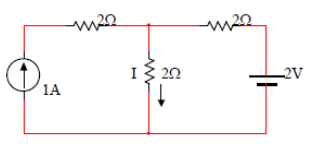A.  1 A

B.  4/3 A

C.  2 A

D.  3 A

Solution:

585.  Find the value of R for which the power supplied by the voltage source is zero.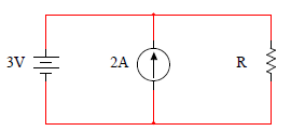A.  0

B.  1.5 ohms

C.  6 ohms

D.  0.667 ohms

Solution:

586.  What  value  of  R  which  ensures  that  the  current through the 60 ohm resistor of this circuit is 1 A?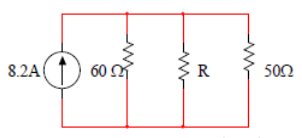A.  5 ohms

B.  10 ohms

C.  15 ohms

D.  20 ohms

Solution:

587.  The current I in the circuit of the figure is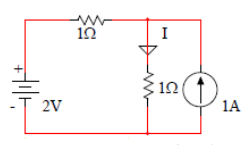A.  2 A

B.  1.5 A

C.  0.5 A

D.  0 A

Solution:

588.  In the circuit shown in the given figure, current I is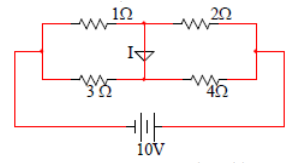A.  –2/5

B.  24/5

C.  18/5

D.  2/5

Solution:

589.  A  35  V  source  is  connected  to  a  series  circuit  of 600Ω  and  R  as  shown.  If  a  voltmeter  of  internal resistance1.2  kΩ  is  connected  across  600  Ω resistor it reads 5 V, find the value of R.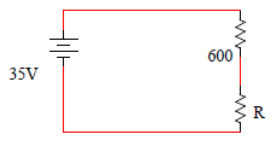A.  1.2 kΩ

B.  2.4 kΩ

C.  3.6 kΩ

D.  7.2 kΩ

Solution:

590.  Find the current in RL in the circuit below.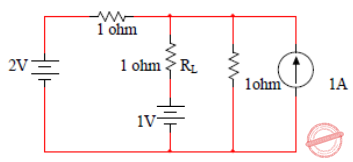A.  0

B.  2/3

C.  1/3

D.  none

Solution:

591.  The  current  flowing  through  the  voltage  source  in the given circuit is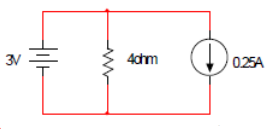A.  1.0 A

B.  0.75 A

C.  0.5 A

D.  0.25 A

Solution:

592.  In the circuit shown, the voltage across 2 Ω resistor is  20  V.  The  5  Ω  resistor  connected  between  the terminals A and B can be replaced by an ideal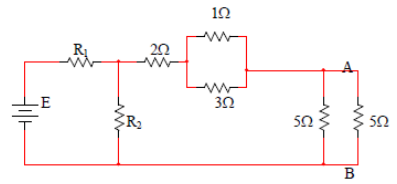A.  Voltage  source  of  25 V  with  +ve  terminal upward

B.  Voltage  source  of  25 V  with  +ve  terminal downward

C.  Current source of 2 A upward

D.  Current source of 2 A downward

Solution:

593.  In  the  circuit  shown  in  the  figure.  The  effective resistance faced by the voltage source is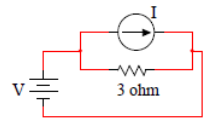A.  1 Ω

B.  2 Ω

C.  3 Ω

D.  3.3 Ω

Solution:

594.  If  a  resistance  ‘R’  of  1Ω  is  connected  across  the terminals  AB  as  shown  in  the  given  fig.  Then  the current flowing through R will be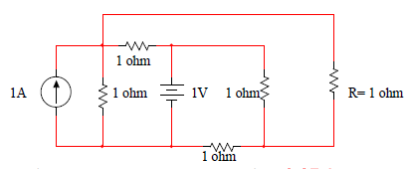A.  1 A

B.  0.5 A

C.  0.25 A

D.  0.125 A

Solution:

595.  Find VL across the ¼ ohm resistor of this circuit.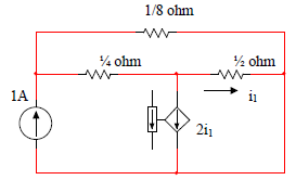A.  1/52 V

B.  2/52 V

C.  3/52 V

D.  5/52 V

Solution:

596.  Find Ix in the fig shown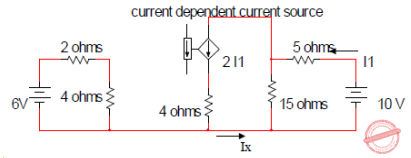A.  1 A

B.  –2 A

C.  2 A

D.  none of these

Solution:

597.  A  particular  resistor  R  dissipates  a  power  of  4 W when  V  alone  is  active.  The  same  resistor  R dissipates a power of 9 watts when I alone is active. The power dissipated by R when both sources are active will be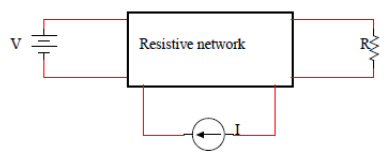A.  1 W

B.  5 W

C.  13 W

D.  25 W

Solution:

598.  The linear network contains only resistors if is1 = 8 A, is2 = 12 A, Vx is found to be 80 v. If is1 = -8 A, is2 = 4 A, Vx = 0 . Find Vx when is1 = is2 = 20 A.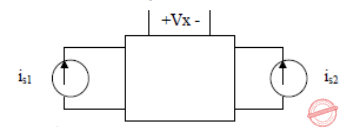A.  –150

B.  150

C.  100

D.  50

Solution:

599.  When R  =  10  ohms,  VR  =  20  V, when R  =  20 ohms VR = 30 V. Find VR when R = 80 ohms.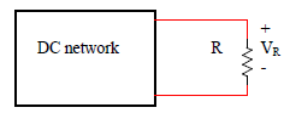A.  40

B.  160

C.  48

D.  none

Solution:

600.  Find V1 & V2.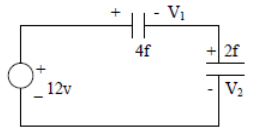A.  4 V, 8 V

B.  8 V, 4 V

C.  6 V, 6 V

D.  12 V, 12 V

Solution:

#### Questions and Answers in DC Circuits

Following is the list of practice exam test questions in this brand new series:

MCQ in DC Circuits
PART 1: MCQ from Number 1 – 50                Answer key: included
PART 2: MCQ from Number 51 – 100            Answer key: included
PART 3: MCQ from Number 101 – 150           Answer key: included
PART 4: MCQ from Number 151 – 200           Answer key: included
PART 5: MCQ from Number 201 – 250           Answer key: included
PART 6: MCQ from Number 251 – 300           Answer key: included
PART 7: MCQ from Number 301 – 350           Answer key: included
PART 8: MCQ from Number 351 – 400            Answer key: included
PART 9: MCQ from Number 401 – 450            Answer key: included
PART 10: MCQ from Number 451 – 500          Answer key: included

P inoyBIX educates thousands of reviewers and students a day in preparation for their board examinations. Also provides professionals with materials for their lectures and practice exams. Help me go forward with the same spirit.

“Will you subscribe today via YOUTUBE?”

Subscribe

PinoyBIX Engineering. © 2014-2020 All Rights Reserved | How to Donate? |